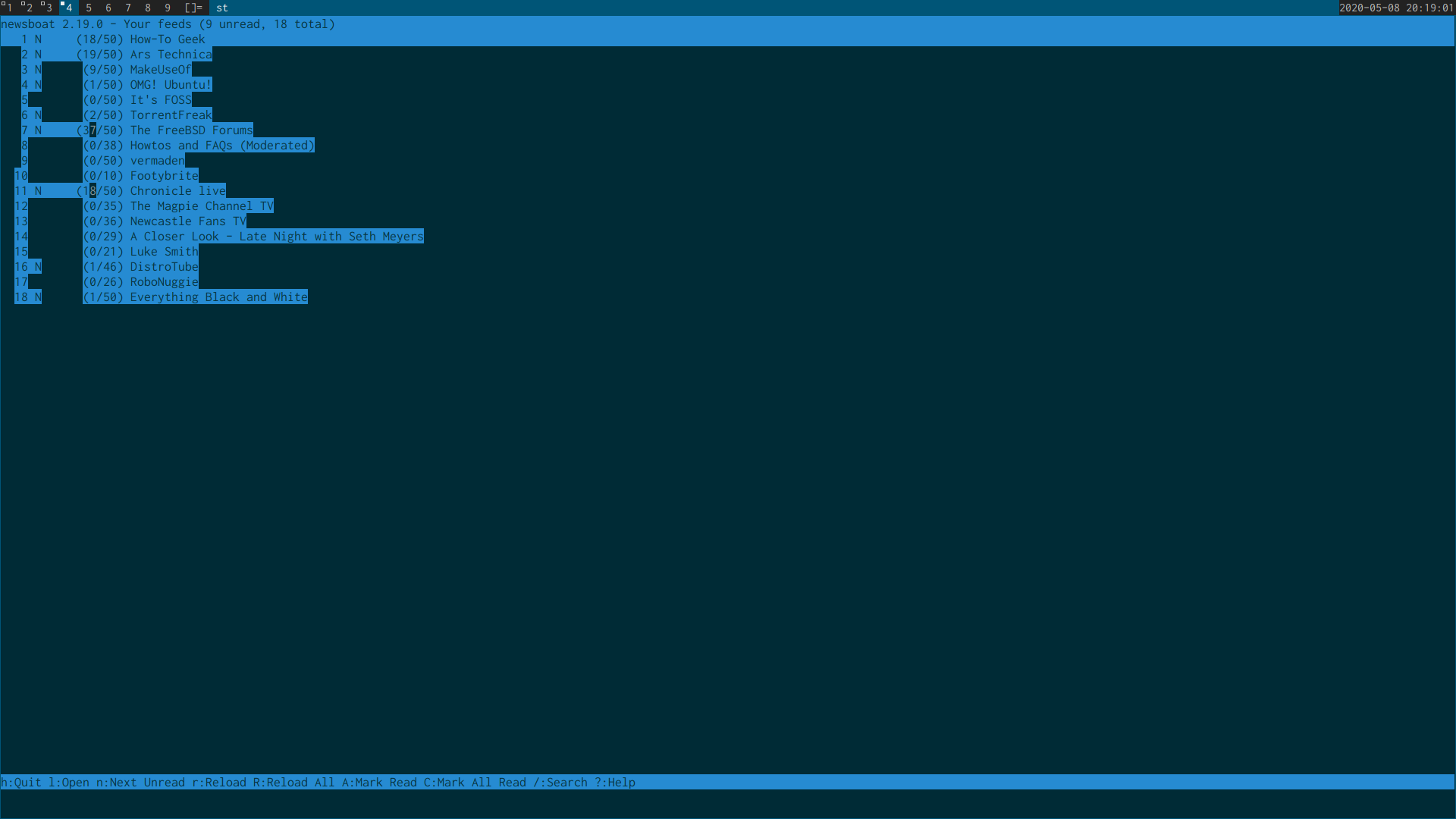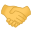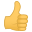# Solvedst terminal convert terminfo to termcap

#### NapoleonWils0n

I built the suckless st terminal from source,
but the makefile use tic to add the st.info file to the terminfo.db which freebsd doesnt use

Freebsd uses termcap.db which means the st terminal has odd behavior especially with ncurses apps like newsboat and ncmpc
because the termcap.db doesnt contain any information about the st terminalI dont get the above issue on ubuntu with the my linux build of the st terminal,
because it uses the terminfo.db

On the sterm freebsd ports page (st is called sterm on freebsd)
there is commit on 20 Mar 2018 17:10:09 which says there are instructions on how to configure terminfo

- Fix the way we handle the terminfo of x11/sterm. FreeBSD 12,
11-STABLE, and 11.2-RELEASE do not require any additional
configuration (thanks to bapt@) . For older versions, there is a
simple set of instructions on how to configure terminfo for x11/sterm
in pkg-message.

So i deleted my installed version of the st terminal and installed the sterm pkg
but looking at the pkg message just return the pkg name
It may the pkg message has been removed

Bash:
`` pkg info -D sterm``

I also had the same issue with ncurses applications with the sterm pkg as i did with my build of st

I did find a post on unix.stackexchange which suggests converting the terminfo to a termcap file using the following command

Bash:
``infocmp -Cr``

i presume you would need that as root and give it the st.info file

Bash:
``# infocmp -Cr st.info``

Has anyone built the st terminal from source
and if so do how did you convert the st.info terminfo file and add it to the termcap.db,
and do you have any issues with ncurses applications

This is the line from the st terminal Makefile which use tic to add the st.info file to the terminfo.db
does anyone know of the correct command for the Makefile to convert st.info file and add to the termcap.db

Bash:
``tic -sx st.info``

Im using urxvt at the moment but the st terminal has better unicode rendering than urxvt,
i also had a look at alacritty but i had the same issue with ncurses applications as i did with the st terminal

alacritty also used about 3.4% cpu doing bugger all compared to the urxvt or st using about 0.2%

The other issue with the st terminal is when you ssh into another machine like a mac for example,
you get double characters printed in the terminal because the mac doesnt have a record of the st terminal in its database,
the fix is you have to start a tmux session on the client machine running the st terminal before you ssh in the remote machine,
that because tmux will change the TERM variable to screen which will be recognized by the machine you are sshing into

i also came across a post that mentioned the st info in the ncurses package is broken,
and hasnt been updated in about 3 years or so

Any terminal recommendations or suggestions

update:
i found this forum post about converting terminfo files

The hard and not yet working 100% way:
1. Install devel/ncurses to get /usr/local/bin/tic
2. Append the x11/sterm related config to /usr/share/misc/termcap by
3. tic -C -t st.info >> termcap. tic can already generate the hashed file but this does not work.
4. Generate the hashed file by cap_mkdb termcap in the termcap directory.
With the hard method something is missing because colored list output does not work and the keys F10 and may be higher are not configured. The termcap entries are limited to 1023bytes or so which is exceeded by the entries in st.info. On the other hand the termcap entries related to other terminals are not really shorter. There is still a lot of stuff to learn for me.

•jpuharic and eldaemon

#### eldaemon

I'm also interested in this as a dwm user. I end up using xterm but prefer st in a few ways.

#### JAW

•memreflect

#### eldaemon

That does sound like the best fix here, although I'm not personally very versed on termcap vs terminfo. Always surprised me how much complexity went into terminals.

#### NapoleonWils0n

I have done a bit more digging and heres what i have come across

running toe which lists the contents of the termcap.db shows that the st terminal and sterm are in the temcap database

Bash:
``toe``

result:

Bash:
``````# Termcap for st terminal taken from the st-0.8 sources
st|simpleterm:\
:am:hs:mi:ms:xn:\
:co#80:it#8:li#24:\
:AL=\E[%dL:DC=\E[%dP:DL=\E[%dM:DO=\E[%dB:IC=\E[%d@:\
:K1=\E[1~:K2=\EOu:K3=\E[5~:K4=\E[4~:K5=\E[6~:LE=\E[%dD:\
:RI=\E[%dC:SF=\E[%dS:UP=\E[%dA:ae=\E(B:al=\E[L:as=\E(0:\
:bl=^G:bt=\E[Z:cd=\E[J:ce=\E[K:cl=\E[H\E[2J:\
:cm=\E[%i%d;%dH:cr=\r:cs=\E[%i%d;%dr:ct=\E[3g:dc=\E[P:\
:dl=\E[M:do=\n:ec=\E[%dX:ei=\E[4l:fs=^G:ho=\E[H:im=\E[4h:\
:is=\E[4l\E>\E[?1034l:k1=\EOP:k2=\EOQ:k3=\EOR:k4=\EOS:\
:k5=\E[15~:k6=\E[17~:k7=\E[18~:k8=\E[19~:k9=\E[20~:\
:kD=\E[3~:kI=\E[2~:kN=\E[6~:kP=\E[5~:kb=\177:kd=\EOB:\
:ke=\E[?1l\E>:kh=\E[1~:kl=\EOD:kr=\EOC:ks=\E[?1h\E=:\
:ku=\EOA:le=^H:mb=\E[5m:md=\E[1m:me=\E[0m:mh=\E[2m:\
:mr=\E[7m:nd=\E[C:rc=\E8:sc=\E7:se=\E[27m:sf=\n:so=\E[7m:\
:sr=\EM:st=\EH:ta=^I:te=\E[?1049l:ti=\E[?1049h:ts=\E]0;:\
:ue=\E[24m:up=\E[A:us=\E[4m:vb=\E[?5h\E[?5l:\
:ve=\E[?12l\E[?25h:vi=\E[?25l:vs=\E[?25h:

st-256color|simpleterm with 256 colors:\
:cc:\
:Co#256:pa#32767:\
:AB=\E[48;5;%dm:AF=\E[38;5;%dm:\
:..Ic=\E]4;%p1%d;rgb\:%p2%{255}%*%{1000}%/%2.2X/%p3%{255}%*%{1000}%/%2.2X/%p4%{255}%*%{1000}%/%2.2X\E\\:\
:oc=\E]104\007:tc=st:

st-meta|simpleterm with meta key:\
:km:\
:is=\E[4l\E>\E[?1034h:mm=\E[?1034h:mo=\E[?1034l:\
:rs=\E[4l\E>\E[?1034h:tc=st:

st-meta-256color|simpleterm with meta key and 256 colors:\
:km:\
:is=\E[4l\E>\E[?1034h:mm=\E[?1034h:mo=\E[?1034l:\
:rs=\E[4l\E>\E[?1034h:tc=st-256color:``````

I installed ncurse because the st terminals Makefile use tic which is installed with ncurses,
ncurses add entries to the termcap.db which is where the st entries came from

I then opened the st terminal and ran infocmp

Bash:
``infocmp``

which outputs this

Bash:
``````#    Reconstructed via infocmp from file: /usr/local/share/misc/terminfo.db
st-256color|stterm-256color|simpleterm with 256 colors,
am, bce, hs, mir, msgr, npc, xenl,
colors#0x100, cols#80, it#8, lines#24, pairs#0x10000,
clear=\E[H\E[2J, cnorm=\E[?12l\E[?25h, cr=\r,
csr=\E[%i%p1%d;%p2%dr, cub=\E[%p1%dD, cub1=^H,
cud=\E[%p1%dB, cud1=\n, cuf=\E[%p1%dC, cuf1=\E[C,
cup=\E[%i%p1%d;%p2%dH, cuu=\E[%p1%dA, cuu1=\E[A,
cvvis=\E[?25h, dch=\E[%p1%dP, dch1=\E[P, dim=\E[2m,
dl=\E[%p1%dM, dl1=\E[M, dsl=\E]0;\007, ech=\E[%p1%dX,
ed=\E[J, el=\E[K, el1=\E[1K, enacs=\E)0,
flash=\E[?5h\$<100/>\E[?5l, fsl=^G, home=\E[H,
hpa=\E[%i%p1%dG, ht=^I, hts=\EH, ich=\E[%p1%d@,
il=\E[%p1%dL, il1=\E[L, ind=\n, indn=\E[%p1%dS, initc@,
invis=\E[8m, is2=\E[4l\E>\E[?1034l, kDC=\E[3;2~,
kEND=\E[1;2F, kHOM=\E[1;2H, kIC=\E[2;2~, kLFT=\E[1;2D,
kNXT=\E[6;2~, kPRV=\E[5;2~, kRIT=\E[1;2C, ka1=\E[1~,
ka3=\E[5~, kb2=\EOu, kbs=^?, kc1=\E[4~, kc3=\E[6~,
kclr=\E[3;5~, kcub1=\EOD, kcud1=\EOB, kcuf1=\EOC,
kcuu1=\EOA, kdch1=\E[3~, kdl1=\E[3;2~, ked=\E[1;5F,
kel=\E[1;2F, kend=\E[4~, kf1=\EOP, kf10=\E[21~,
kf11=\E[23~, kf12=\E[24~, kf13=\E[1;2P, kf14=\E[1;2Q,
kf15=\E[1;2R, kf16=\E[1;2S, kf17=\E[15;2~, kf18=\E[17;2~,
kf19=\E[18;2~, kf2=\EOQ, kf20=\E[19;2~, kf21=\E[20;2~,
kf22=\E[21;2~, kf23=\E[23;2~, kf24=\E[24;2~,
kf25=\E[1;5P, kf26=\E[1;5Q, kf27=\E[1;5R, kf28=\E[1;5S,
kf29=\E[15;5~, kf3=\EOR, kf30=\E[17;5~, kf31=\E[18;5~,
kf32=\E[19;5~, kf33=\E[20;5~, kf34=\E[21;5~,
kf35=\E[23;5~, kf36=\E[24;5~, kf37=\E[1;6P, kf38=\E[1;6Q,
kf39=\E[1;6R, kf4=\EOS, kf40=\E[1;6S, kf41=\E[15;6~,
kf42=\E[17;6~, kf43=\E[18;6~, kf44=\E[19;6~,
kf45=\E[20;6~, kf46=\E[21;6~, kf47=\E[23;6~,
kf48=\E[24;6~, kf49=\E[1;3P, kf5=\E[15~, kf50=\E[1;3Q,
kf51=\E[1;3R, kf52=\E[1;3S, kf53=\E[15;3~, kf54=\E[17;3~,
kf55=\E[18;3~, kf56=\E[19;3~, kf57=\E[20;3~,
kf58=\E[21;3~, kf59=\E[23;3~, kf6=\E[17~, kf60=\E[24;3~,
kf61=\E[1;4P, kf62=\E[1;4Q, kf63=\E[1;4R, kf7=\E[18~,
kf8=\E[19~, kf9=\E[20~, khome=\E[1~, kich1=\E[2~,
kil1=\E[2;5~, kind=\E[1;2B, kmous=\E[M, knp=\E[6~,
kpp=\E[5~, kri=\E[1;2A, krmir=\E[2;2~, mc0=\E[i, mc4=\E[4i,
mc5=\E[5i, oc@, op=\E[39;49m, rc=\E8, rev=\E[7m, ri=\EM,
ritm=\E[23m, rmacs=\E(B, rmcup=\E[?1049l, rmir=\E[4l,
rmkx=\E[?1l\E>, rmso=\E[27m, rmul=\E[24m, rs1=\Ec,
rs2=\E[4l\E>\E[?1034l, sc=\E7,
setab=\E[%?%p1%{8}%<%t4%p1%d%e%p1%{16}%<%t10%p1%{8}%-%d%e48;5;%p1%d%;m,
setaf=\E[%?%p1%{8}%<%t3%p1%d%e%p1%{16}%<%t9%p1%{8}%-%d%e38;5;%p1%d%;m,
sgr=%?%p9%t\E(0%e\E(B%;\E[0%?%p6%t;1%;%?%p2%t;4%;%?%p1%p3%|%t;7%;%?%p4%t;5%;%?%p5%t;2%;%?%p7%t;8%;m,
sgr0=\E[0m, sitm=\E[3m, smacs=\E(0, smcup=\E[?1049h,
smir=\E[4h, smkx=\E[?1h\E=, smso=\E[7m, smul=\E[4m,
tbc=\E[3g, tsl=\E]0;, u6=\E[%i%d;%dR, u7=\E[6n,
u8=\E[?1;2c, u9=\E[c, vpa=\E[%i%p1%dd,``````

So i decided to use infotocap on the st.info file and compare the results to the termcap.db

Bash:
``infotocap``

which outputs this error message
Bash:
``````"st.info", line 189, col 20, terminal 'st': unknown capability 'rmxx'
"st.info", line 190, col 19, terminal 'st': unknown capability 'smxx'
"st.info", line 192, col 11, terminal 'st': unknown capability 'Tc'
"st.info", line 193, col 33, terminal 'st': unknown capability 'Ms'
"st.info", line 194, col 18, terminal 'st': unknown capability 'Se'
"st.info", line 195, col 22, terminal 'st': unknown capability 'Ss'``````

and then this code which is exactly the same as the entries in the termcap file

Bash:
``````# (untranslatable capabilities removed to fit entry within 1023 bytes)
# (sgr removed to fit entry within 1023 bytes)
# (acsc removed to fit entry within 1023 bytes)
# (terminfo-only capabilities suppressed to fit entry within 1023 bytes)
st| simpleterm:\
:am:hs:mi:ms:xn:\
:co#80:it#8:li#24:\
:AL=\E[%dL:DC=\E[%dP:DL=\E[%dM:DO=\E[%dB:IC=\E[%d@:\
:K1=\E[1~:K2=\EOu:K3=\E[5~:K4=\E[4~:K5=\E[6~:LE=\E[%dD:\
:RI=\E[%dC:SF=\E[%dS:UP=\E[%dA:ae=\E(B:al=\E[L:as=\E(0:\
:bl=^G:bt=\E[Z:cd=\E[J:ce=\E[K:cl=\E[H\E[2J:\
:cm=\E[%i%d;%dH:cr=\r:cs=\E[%i%d;%dr:ct=\E[3g:dc=\E[P:\
:dl=\E[M:do=\n:ec=\E[%dX:ei=\E[4l:fs=^G:ho=\E[H:im=\E[4h:\
:is=\E[4l\E>\E[?1034l:k1=\EOP:k2=\EOQ:k3=\EOR:k4=\EOS:\
:k5=\E[15~:k6=\E[17~:k7=\E[18~:k8=\E[19~:k9=\E[20~:\
:kD=\E[3~:kI=\E[2~:kN=\E[6~:kP=\E[5~:kb=\177:kd=\EOB:\
:ke=\E[?1l\E>:kh=\E[1~:kl=\EOD:kr=\EOC:ks=\E[?1h\E=:\
:ku=\EOA:le=^H:mb=\E[5m:md=\E[1m:me=\E[0m:mh=\E[2m:\
:mr=\E[7m:nd=\E[C:rc=\E8:sc=\E7:se=\E[27m:sf=\n:so=\E[7m:\
:sr=\EM:st=\EH:ta=^I:te=\E[?1049l:ti=\E[?1049h:ts=\E]0;:\
:ue=\E[24m:up=\E[A:us=\E[4m:vb=\E[?5h\E[?5l:\
:ve=\E[?12l\E[?25h:vi=\E[?25l:vs=\E[?25h:

st-256color| simpleterm with 256 colors:\
:cc:\
:Co#256:pa#32767:\
:AB=\E[48;5;%dm:AF=\E[38;5;%dm:\
:..Ic=\E]4;%p1%d;rgb\:%p2%{255}%*%{1000}%/%2.2X/%p3%{255}%*%{1000}%/%2.2X/%p4%{255}%*%{1000}%/%2.2X\E\\:\
:oc=\E]104\007:tc=st:

st-meta| simpleterm with meta key:\
:km:\
:is=\E[4l\E>\E[?1034h:mm=\E[?1034h:mo=\E[?1034l:\
:rs=\E[4l\E>\E[?1034h:tc=st:

st-meta-256color| simpleterm with meta key and 256 colors:\
:km:\
:is=\E[4l\E>\E[?1034h:mm=\E[?1034h:mo=\E[?1034l:\
:rs=\E[4l\E>\E[?1034h:tc=st-256color:``````

So im guesing the unknown capability errors when converting the terminfo file to termcap might be the issue

Strange thing is i only have an issue the st terminal and ncurses applications like newsboat, ncmpc and weechat
which make me wonder if the issue is with the ncurses package and the termcap.db perhaps missing something
that causes the st terminal to have odd behaviour with ncurses applications

I have the exact same set up on ubuntu and the st terminal doesnt have any issues with ncurses applications,
and the only difference is linux is using terminfo whereas freebsd is using termcap

so my hunch is converting the st.info terminfo file to termcap format is the issue
and something is being missed out in the conversion process which causes the issue with ncurses applications

The unknown capability errors when converting the st.info file with infotocmp relate to these lines in the st.info file

Bash:
``````# XTerm extensions
rmxx=\E[29m,
smxx=\E[9m,
# tmux extensions, see TERMINFO EXTENSIONS in tmux(1)
Tc,
Ms=\E]52;%p1%s;%p2%s\007,
Se=\E[2 q,
Ss=\E[%p1%d q,``````

So the long and short is installing ncurses seems to add the st terminal definitions to the termcap.db,
and definitions in the termcap are exactly the same as when you run infotocmp on the st.info file

I thought id post the results of my digging around in case anyone can spot what the issue might be

The alternative is to wait until Freebsd switches from using termcap to terminfo,
and im not holding my breath on that one because i wouldnt have thought that kind of change would happen quickly if at all

update:

i also tried copying the /usr/share/misc/termcap to the Desktop
commenting out the st terminal definations

then i used infotocap to convert the st.info file into a termcap file
and added the st termcap to the system termcap i copied to the desktop

after that i converted the termcap to a termcap.db file

Bash:
``cap_mkdb -f termcap termcap``

which created a termcap.db file which i then copied to ~/.termcap.db
i then logged out and back in again and the ~/.termcap.db then override the system termcap.db file

but the terminal definations produced by converting the st.info file are exactly the same as the ones in the system termcap
so i still have the same issue with the st terminal and ncurses applications not working properly

if i run tmux with the st terminal which changes the TERM variable to screen and then start an ncurses application
it still has some issues with background colours not being set properly but not as many issue as when using st without tmux
in which case the term variable would be set to st-256color

it looks like its the st-256color terminal definition in termcap that is the issue
and if you convert the st.info file using infotocap you get exactly same terminal definitions already in the system termcap

setting the TERM to xterm-256color fixes the issue with st terminal not displaying colours or redrawing the screen with newsboat
which is an ncurses based but not with ncmpc which will randomly highlight text or the text disappears when you scroll

you can either set the term in the st config.h file which would be the best solution,
or you could set the TERM in your shell config file like you bashrc or zshrc,
but generally its not good practice to set the TERM variable

changing the st term to xterm-256color does also produce some issue like the screen not clearing properly

••jpuharic and eldaemon

#### memreflect

Strange thing is i only have an issue the st terminal and ncurses applications like newsboat, ncmpc and weechat
which make me wonder if the issue is with the ncurses package and the termcap.db perhaps missing something
that causes the st terminal to have odd behaviour with ncurses applications
What is the output of ` ldd /usr/local/bin/ncmpc | grep -e curses -e tinfo `? If you're linking against devel/ncurses you should see an entry for libtinfo.so.6 or libtinfow.so.6; you should also see libncurses.so.6 or libncursesw.so.6 if any ncurses entries are listed, and there should only be one ncurses entry.

Your initial post suggests that you are building the ports using the usual ` make install`, so the port should link against devel/ncurses rather than the base system's ncurses lib, assuming devel/ncurses was installed first. In theory, this means everything should work pretty much the same as in Linux because terminfo is consulted before termcap, except when using programs like sh(1) in the base system that only know about termcap.

However, it's possible that something is broken somewhere. I know ports-mgmt/dialog4ports (used for configuring port options) looked terrible when I experimentally tried to replace the base system's curses library with the libs from devel/ncurses and add terminfo support to the entire base system by compiling it from source. Some text even blinks in xterm when there shouldn't be any blinking occurring, and there are certain parts of the dialog window that look similar to your screenshot. Everything else appeared to be working, however, so I assumed it was the port's fault. But maybe it's a problem with devel/ncurses or a terminfo/termcap incompatibility somewhere.

#### NapoleonWils0n

im not using ports,

#### memreflect

im not using ports,
If you're not using ports, then audio/ncmpc and the other programs you're using are likely linked against the base system's ncurses libs in the pre-built binary packages, meaning terminfo won't be available to use the full terminal description of ` st`. The ldd(1) command I posted will verify that for you by listing something like
Code:
``libncurses.so.8 => /lib/libncurses.so.8``

#### NapoleonWils0n

this is the output i get with ldd

Bash:
``libncursesw.so.8 => /lib/libncursesw.so.8 (0x80036a000)``

i seem to have libncursesw.so.8 and not libncurses.so.8

also i dont have a terminfo file only a termcap and termcap.db,
even though the st's Makefile uses tc to add entries to the terminfo file

Bash:
``tic -sx st.info``

i had to install the ncurses pkg because the st Makefile uses tic

looking in /lib i can see i have
Bash:
``libncurses.so.8   libncursesw.so.8``

#### memreflect

this is the output i get with ldd

Bash:
``libncursesw.so.8 => /lib/libncursesw.so.8 (0x80036a000)``

i seem to have libncursesw.so.8 and not libncurses.so.8

Yes, that's still the base system's curses library. Unfortunately, it seems you're stuck with the limited termcap capabilities if you decide to stick with st. Does xterm work any better?

also i dont have a terminfo file only a termcap and termcap.db,
even though the st's Makefile uses tc to add entries to the terminfo file

Bash:
``tic -sx st.info``
When you install devel/ncurses, it will install /usr/local/share/misc/terminfo.db and ` tic`, which ` st` uses to install the terminfo description of its terminal capabilities. However, programs that don't rely on devel/ncurses, such as the audio/ncmpc package you installed using pkg(8) won't even look at the terminfo database, so the termcap description of ` st` will be used instead, which means there's nothing you can do in this situation.

I wish I had better news, but that's just how things go sometimes.

•jpuharic

#### NapoleonWils0n

memreflect thanks for the explanation that clears a few things up
makes perfect sense

xterm and rxvt dont have any issues with ncurses applications,
its just the st terminal that has the issue but only on freebsd and not linux

i also came across the same issue with alacritty as well on freebsd
was just trying a few rxvt alternatives

not entirely wasted time as i learnt a few things along the way

ill stick with rxvt at least it works without any issues apart from sometimes not rendering unicode characters